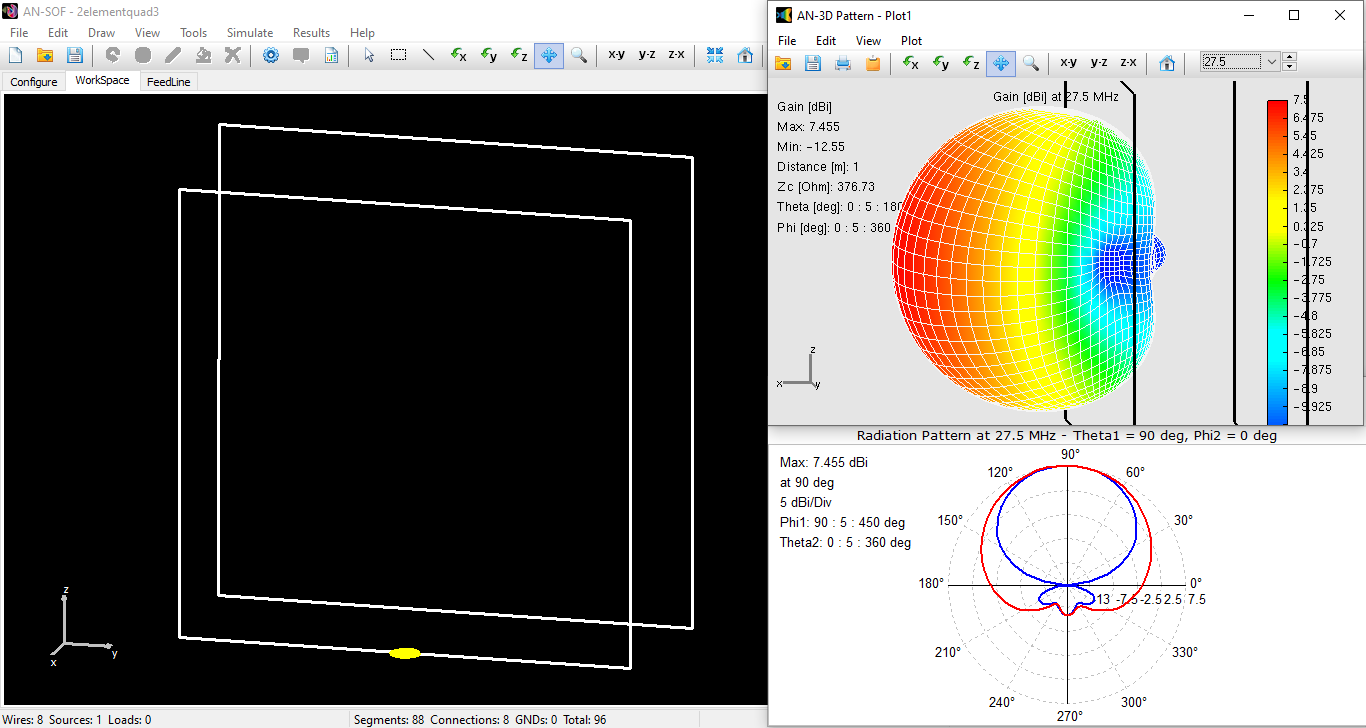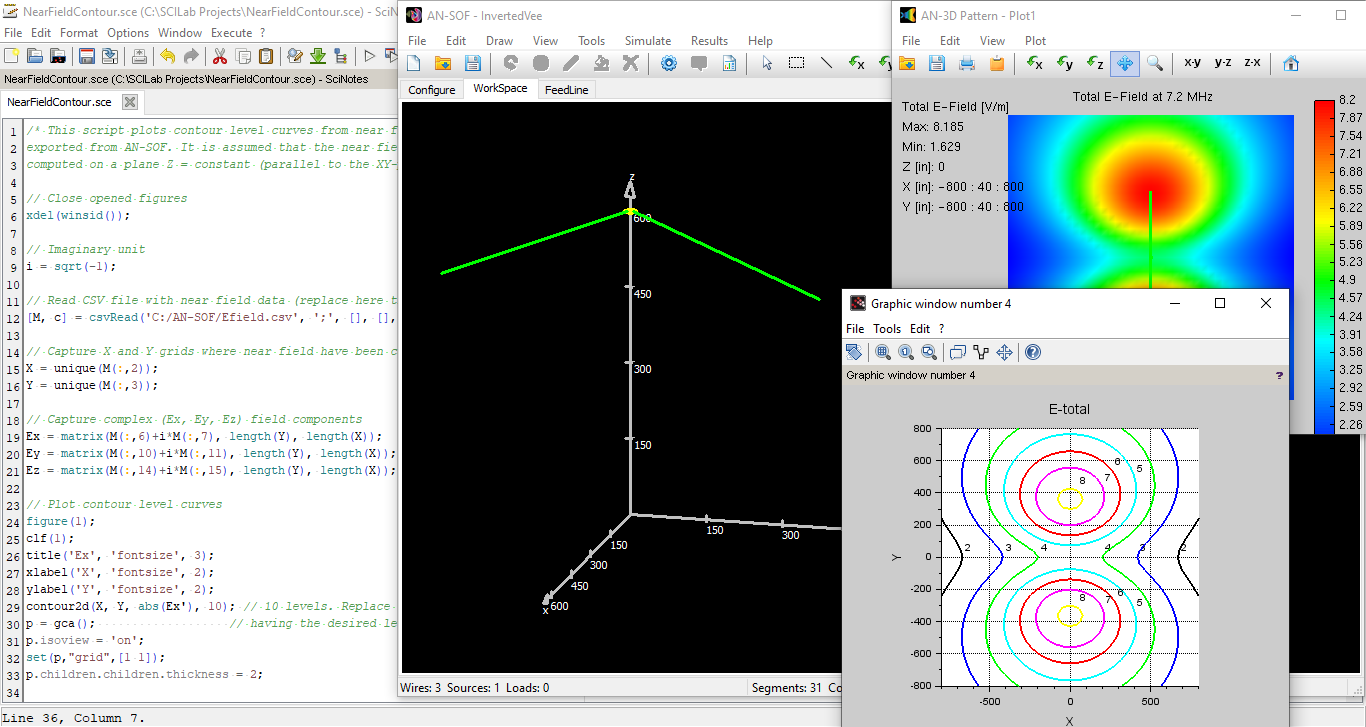# Category -Examples

Examples of antenna models and wire structures in general.

## Cylindrical Antenna

A center-fed cylindrical antenna is the simplest example that we can simulate. It consists of a straight wire with a source at its center and becomes a half-wave dipole when the frequency is such that the length of the antenna is half the wavelength.

## Monopole Over Real Ground

A monopole is a vertical element connected to a ground plane and with the feed point at its base. In this example we will simulate a radio mast on an imperfect ground, which is used for broadcasting in the LF and MF bands.

## Helix Antenna in Axial Mode

The helix is a good example where we need curved segments to describe the geometry of the antenna. When the length of the helix is of the order of or greater than the wavelength, it can work in the so-called "axial mode".

## Loop Antenna

A good example where we need curved segments to model an antenna is the circular loop case. When the loop is small compared to the wavelength, the radiation resistance is proportional to the square of the loop area.

## A Transmission Line

Two-wire transmission lines can be modeled explicitly in AN-SOF. In this example, the line will have a single wire but there will be a ground plane below it, so we have the mirror image of the wire as the return of the line.

## An RLC Circuit

The ability of AN-SOF to simulate at extremely low frequencies can be demonstrated with a model of an RLC circuit that will resonate at only 800 Hz, so the wavelength is 375 km!## Dipole Antenna

Frequency sweep simulation of a cylindrical dipole antenna. The results show how the current distribution along the wire approaches a sine function.## Half-Wave Dipole

Center-fed half-wave dipole antenna at 300 MHz. The wavelength is close to 1 meter, so the dipole length equals 0.5 meters.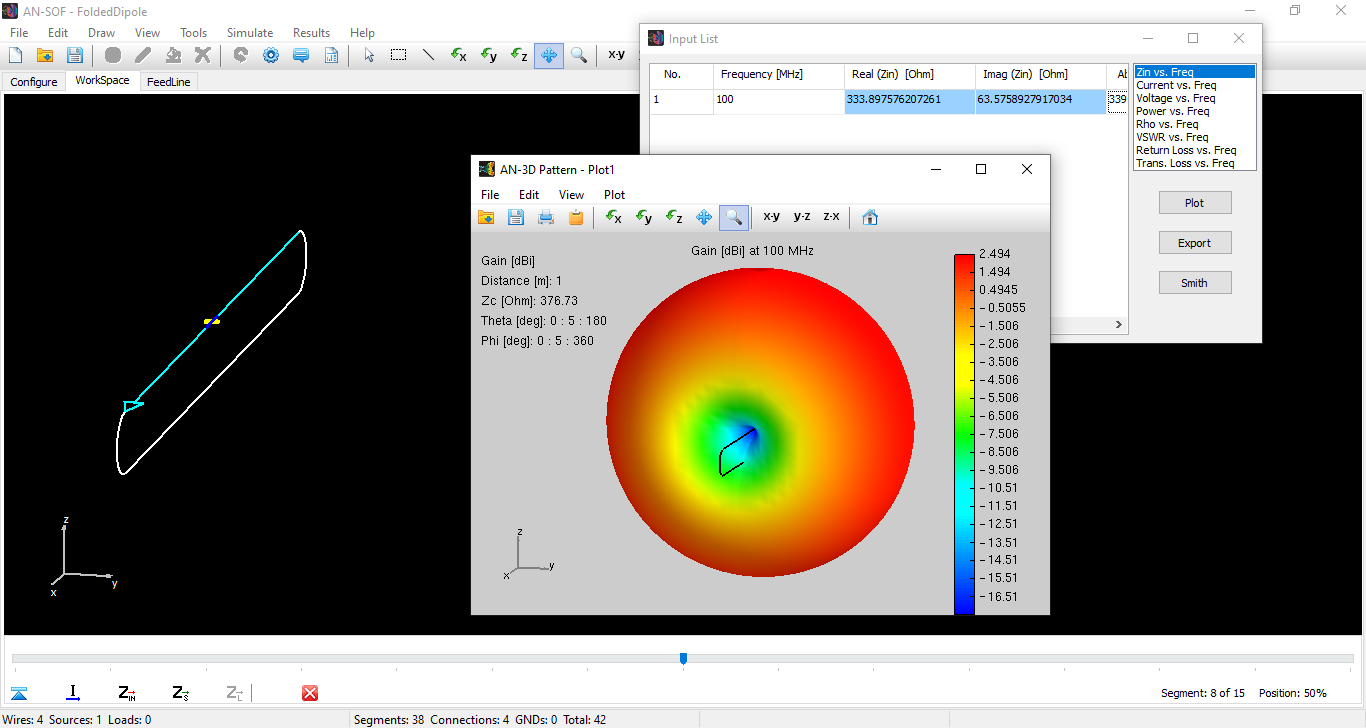## Folded Dipole

Simulation of a folded dipole using curved wires at the dipole ends. The curved part is modeled exactly using conformal segments.The antenna is composed of four vertical monopoles over ground. Each monopole is fed at its base by a voltage source of the same amplitude and phase as the others.## Monopole Above Earth Ground

The monopole is used for AM (Amplitude Modulation) radio transmissions. The far-field radiation pattern in the Fraunhofer zone is distorted due to the finite conductivity of the soil.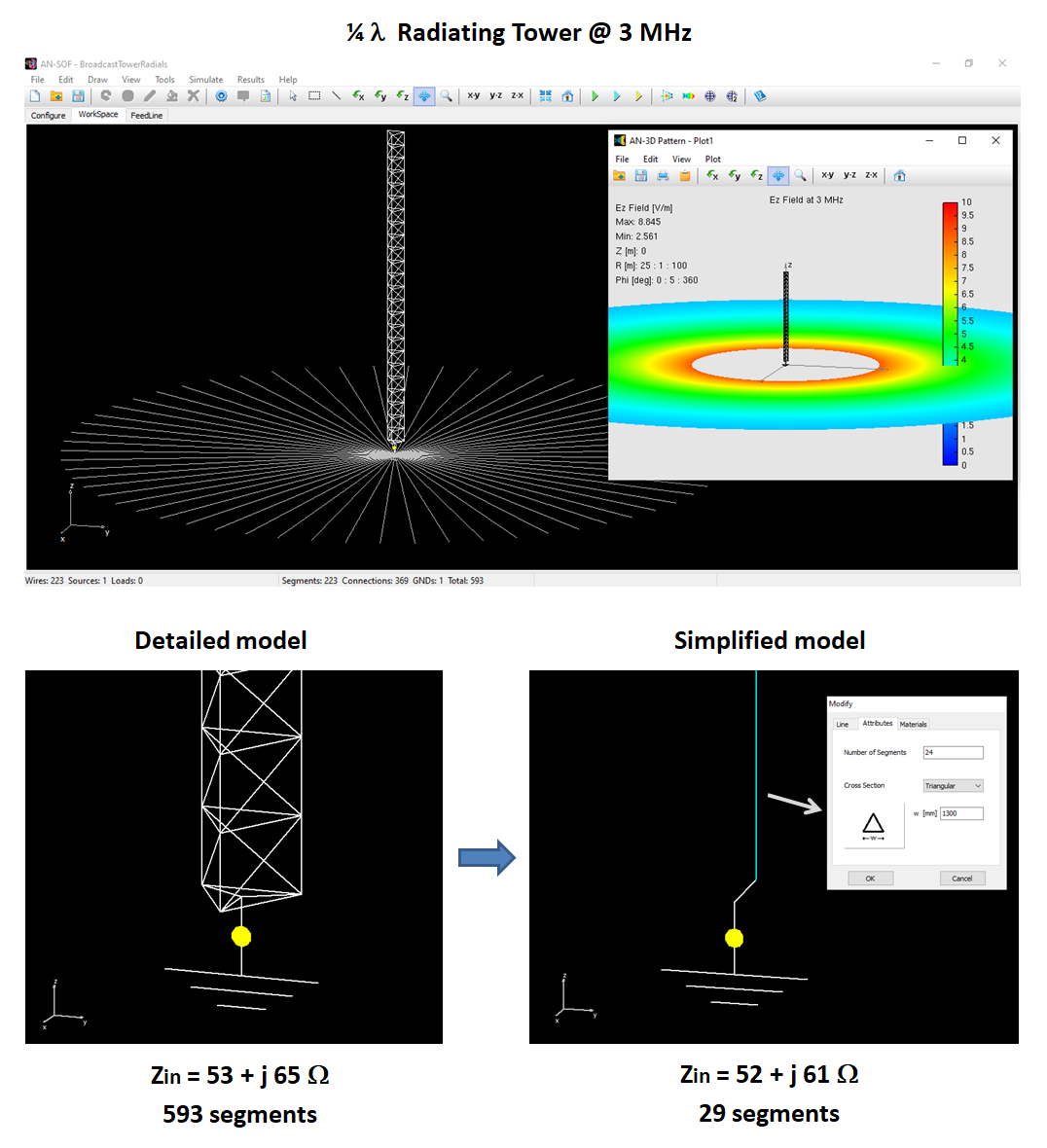## Radio Mast Above Wire Screen

Radiating towers or radio masts can be modeled in AN-SOF with a high degree of detail. This example shows a quarter-wave monopole antenna connected to a radial wire ground screen on a real ground plane.## Square Loop Antenna

The total length of the loop is about 0.4 wavelengths, so the current distribution shows a semi-cycle of a sine function.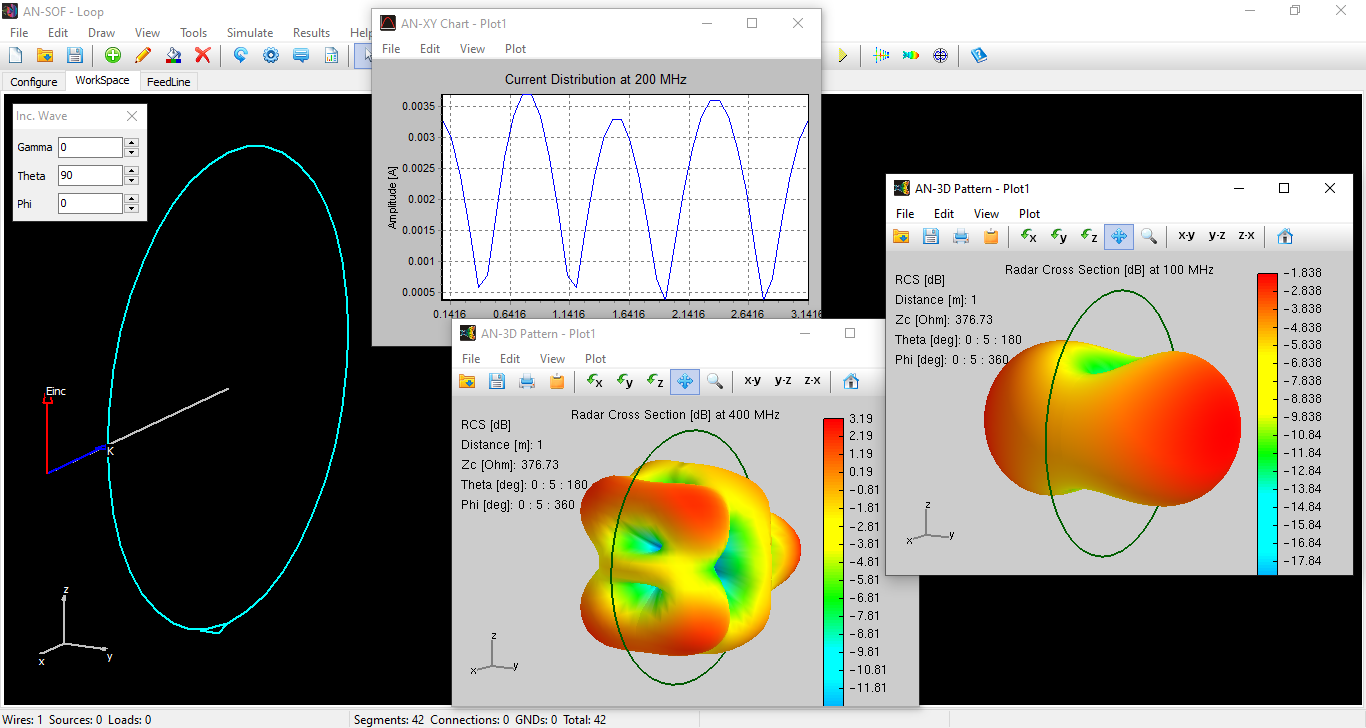## Receiving Loop Antenna

Frequency sweep simulation of a receiving circular loop antenna. The loop is modeled using conformal segments, which exactly follow the contour of the antenna geometry.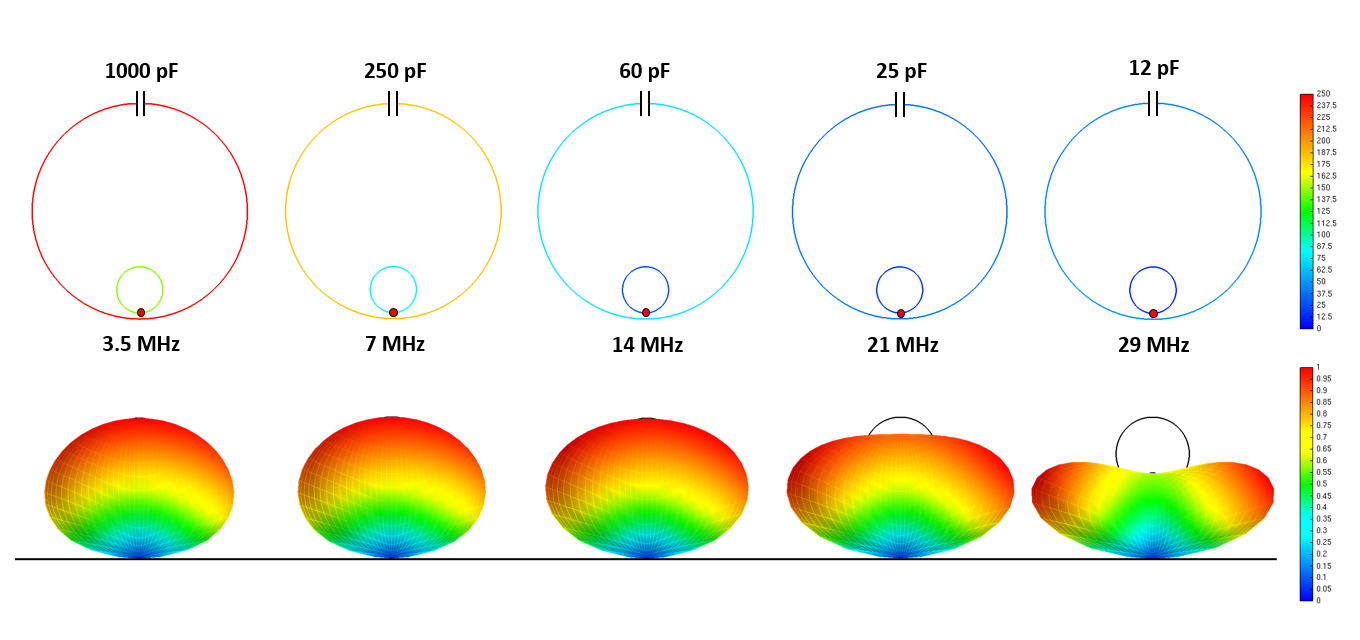## Magnetic Loop Antenna

This small transmitting loop antenna is 1 meter high above average ground, so the radiation pattern is not donut-shaped as expected for a small loop in free space. The value of the tuning capacitor is indicated for each resonance frequency.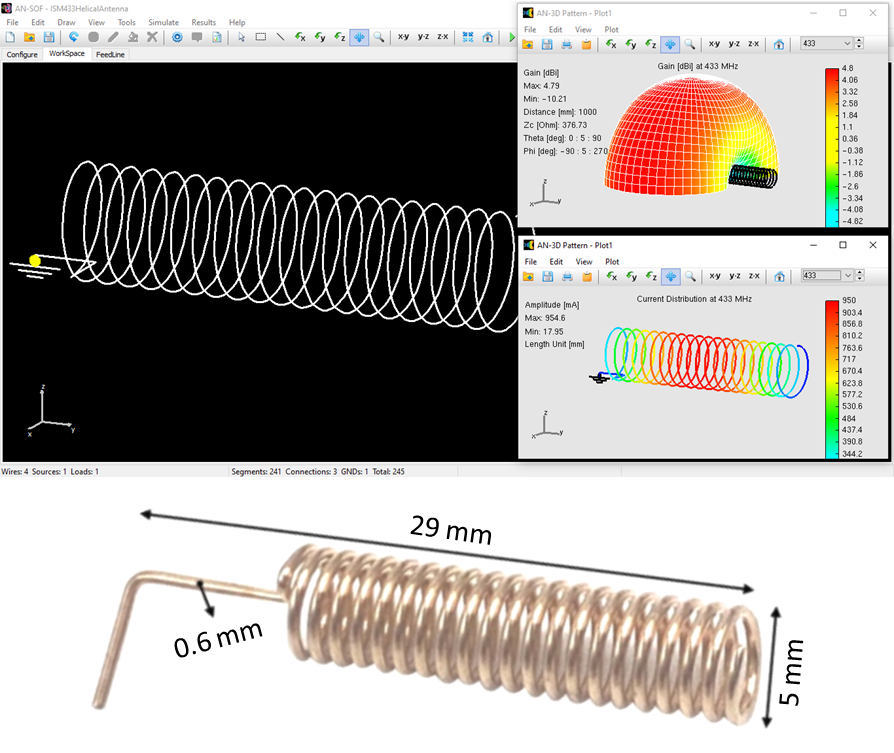## ISM 433MHz Helical Antenna

Small helical antennas for the 433 MHz ISM band are a good example where we need advanced software that has the ability to model curved wires with exact description of the helix contour.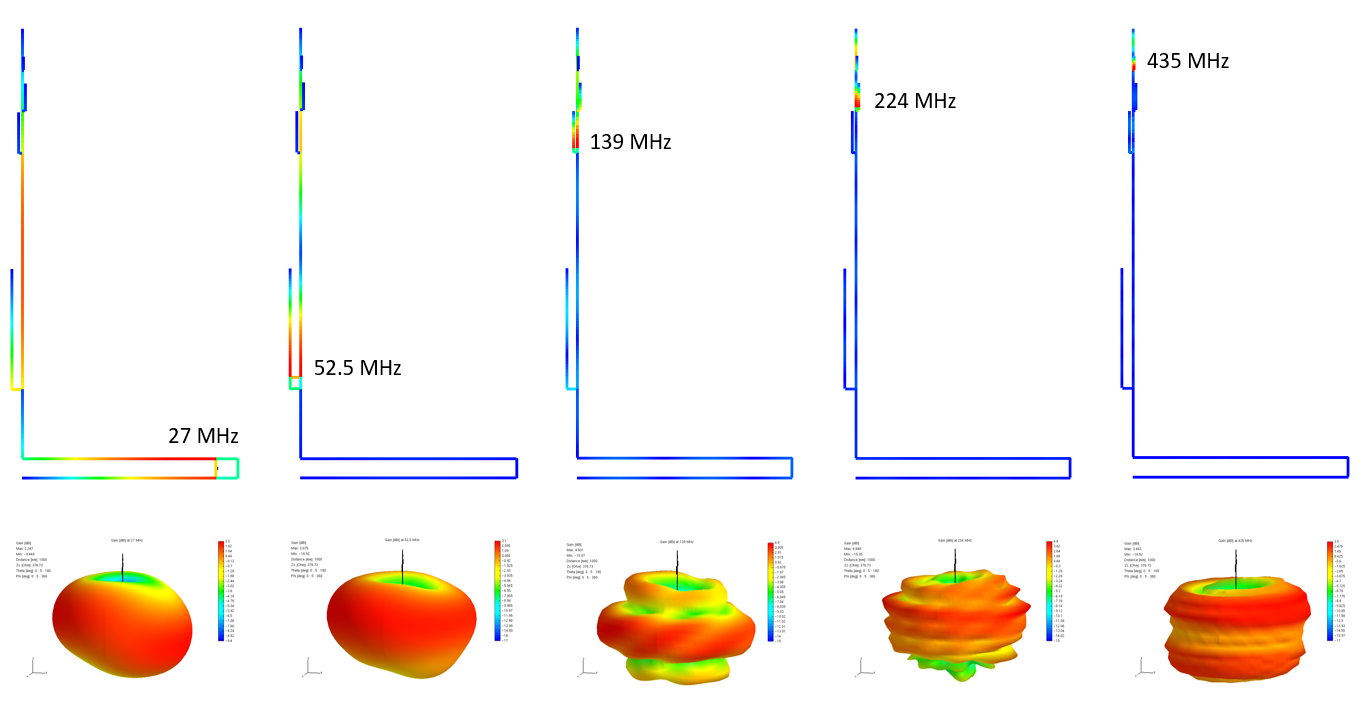## Multiband J-Pole Antenna

This design is for 5 bands on a single pole. Each "J" corresponds to a band and therefore the feed point changes depending on the frequency.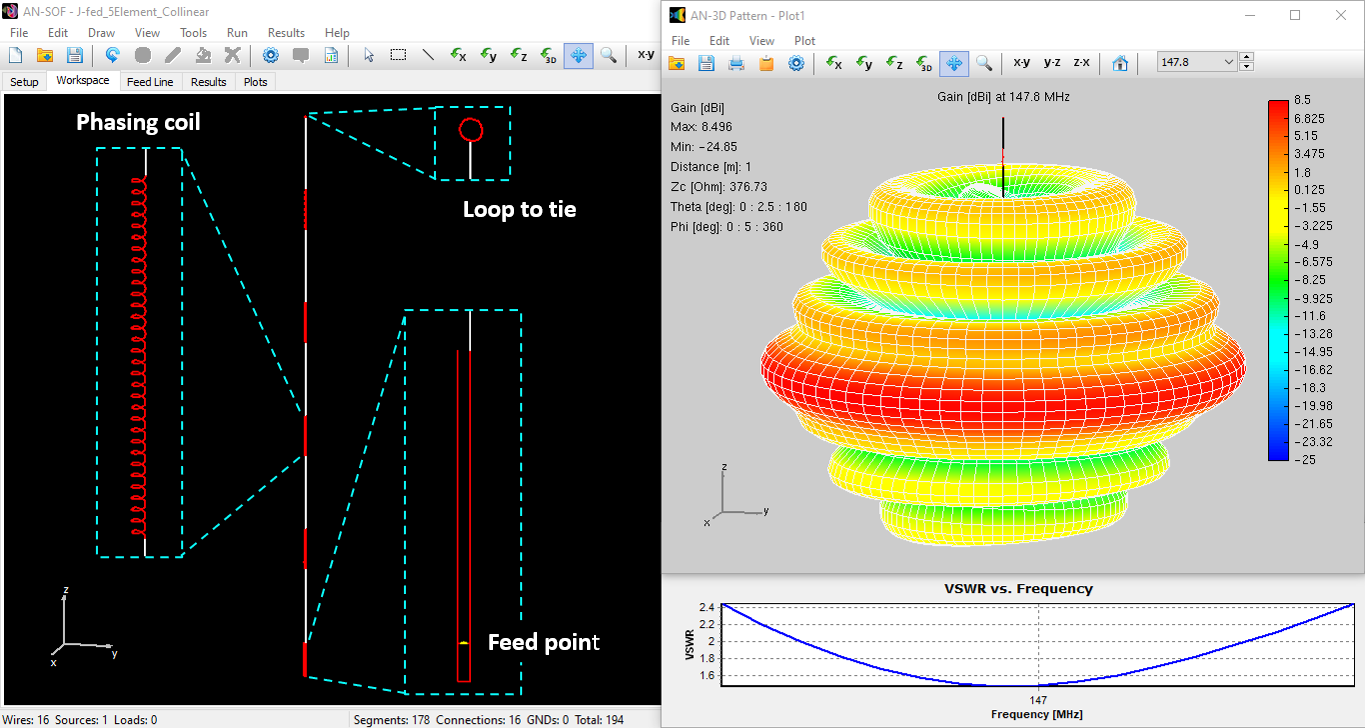## Modeling a J-Fed 5-Element Collinear Antenna for the 2 m Band

If you're looking for a high-performing omnidirectional antenna, consider this design. It boasts 5 collinear radiant elements that are connected by phasing coils.## 3-Element Yagi-Uda

Simulation of a Yagi antenna that consists of three linear wires. A directional radiation pattern is obtained as can be expected.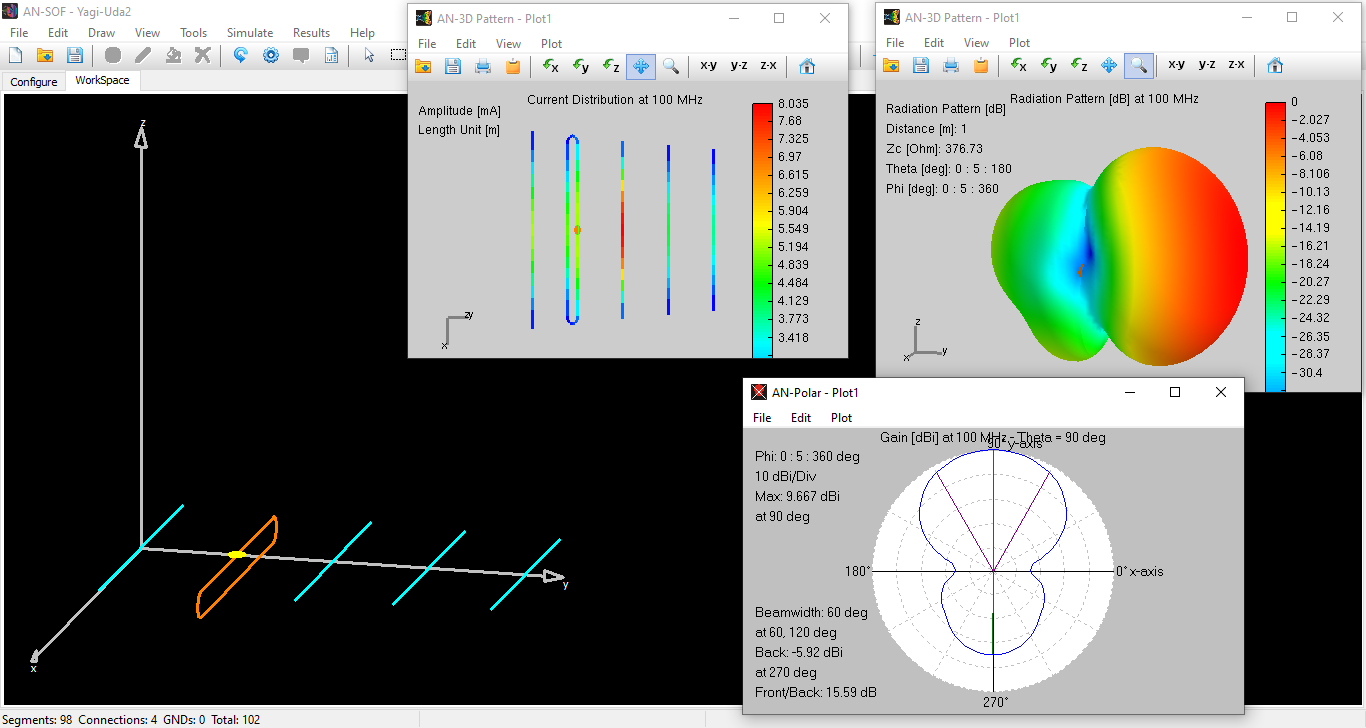## 5-Element Yagi-Uda

Simulation of a Yagi antenna that consists of five linear wires. The driven element is a folded dipole which does not change the radiation pattern shape, but it changes the input impedance for an easier impedance matching.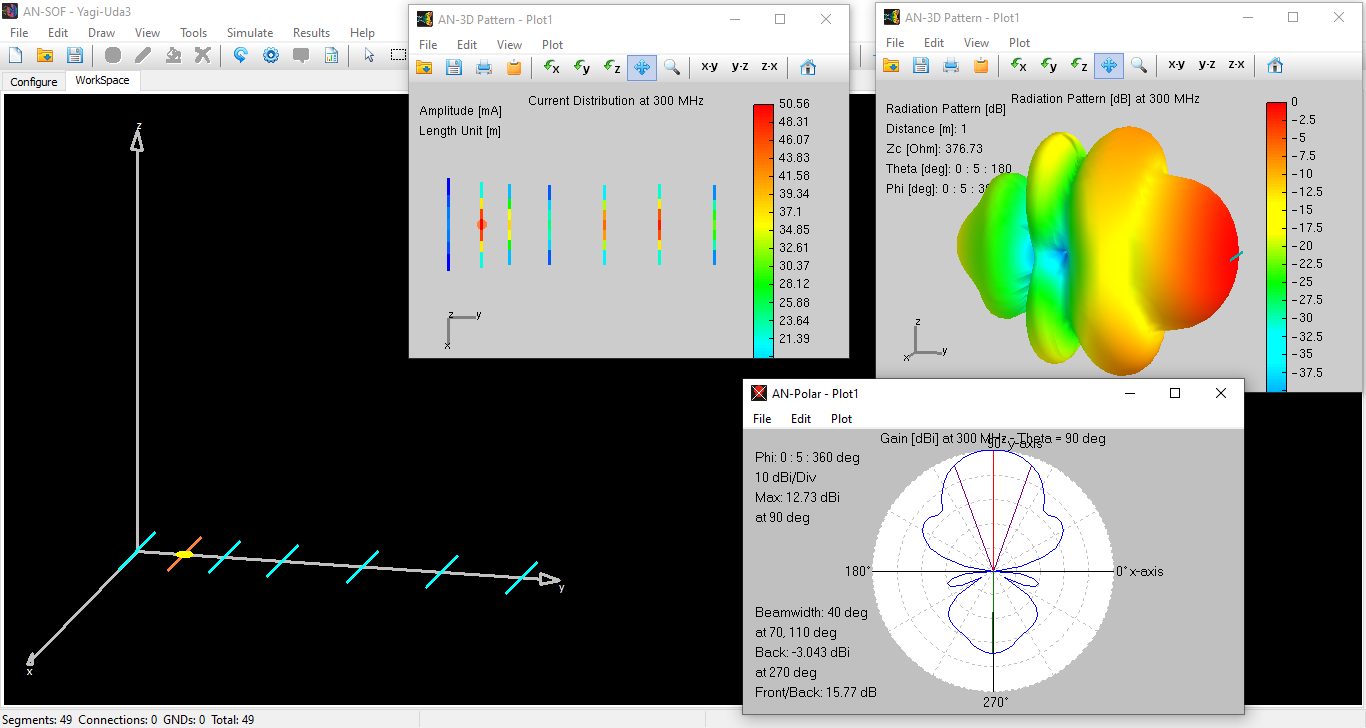## 7-Element Yagi-Uda

Simulation of a Yagi antenna that consists of seven linear wires. A directional radiation pattern is obtained as can be expected.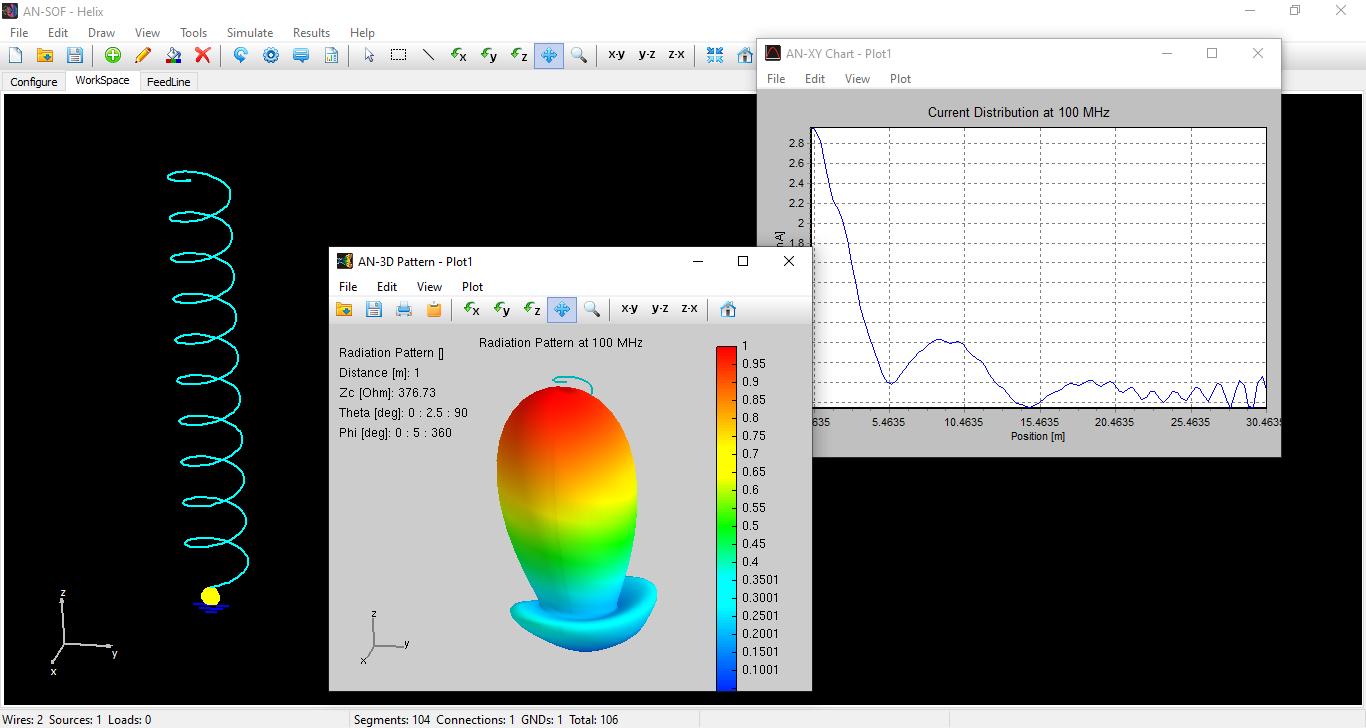## Helical Antenna with PEC Reflector

Directional helix antenna backed by a perfect ground plane. A directional radiation pattern is obtained pointing towards the helix axis.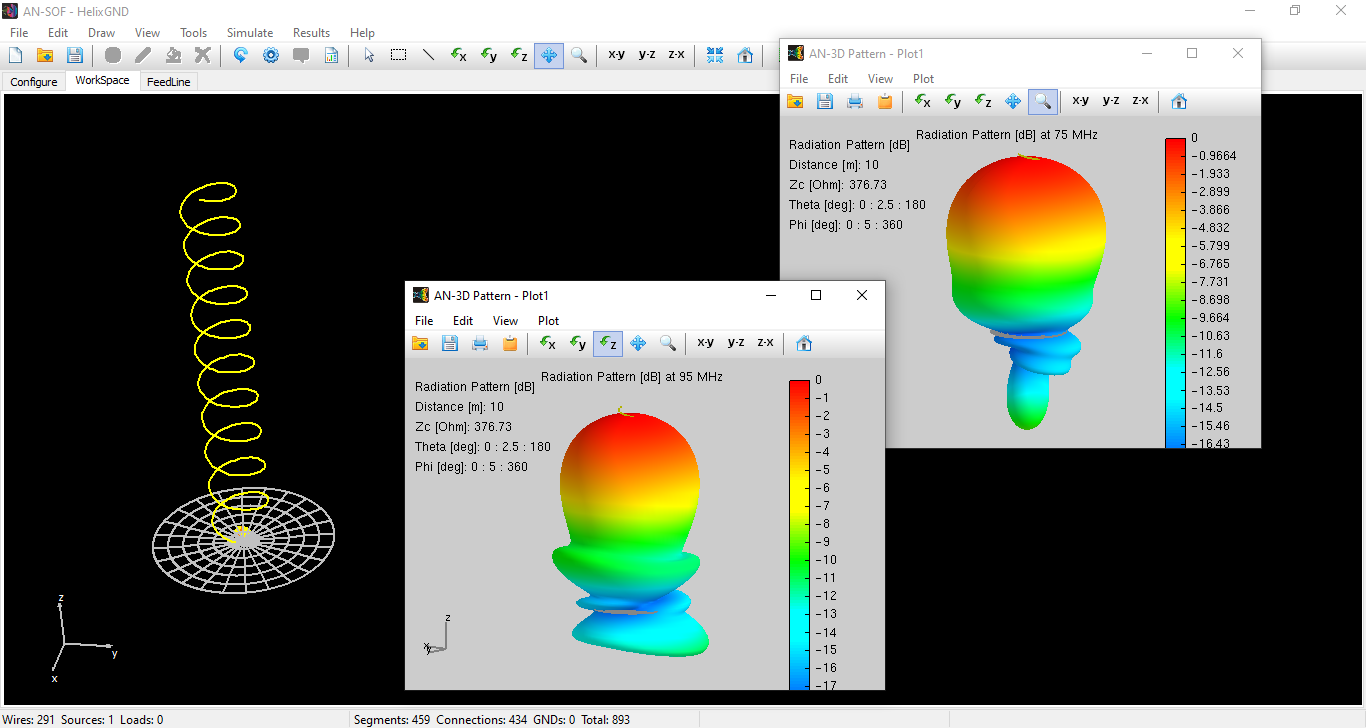## Helical Antenna with Grid Reflector

Frequency sweep simulation of a directional helix antenna backed by a ground plane of finite size. The ground plane is modeled using a circular grid of thin wires.## QFH Antenna for NOAA Satellite Reception

This Quadrifilar Helix (QFH) antenna, also known as QHA, can be used for signal reception from NOAA satellites. These are omnidirectional antennas made up of 4 wires with a helical shape.## Directional V Antenna

The horizontal arms of the V-antenna are 3 wavelengths long, so six semi-cycles of a sine function can be seen as a current distribution along the arms.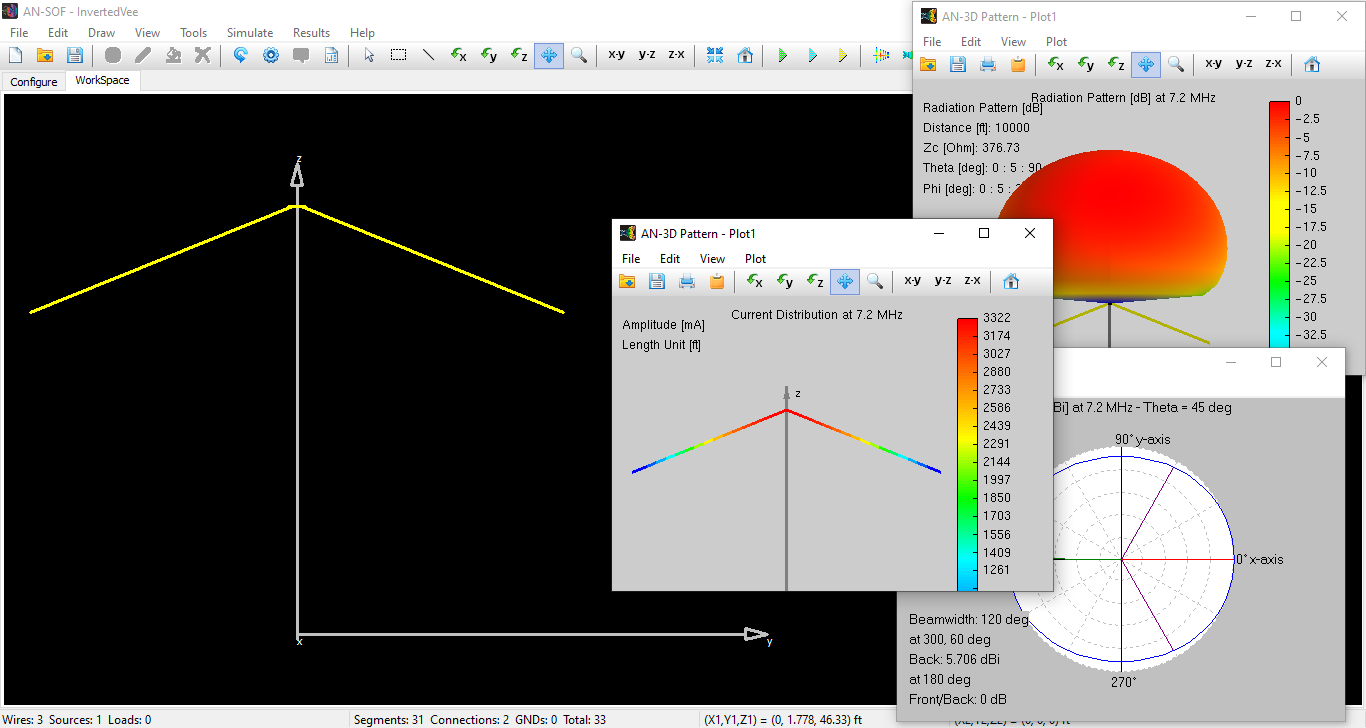## Inverted V Antenna

Inverted V antenna over real ground. The operating frequency is 7.2 MHz which corresponds to a wavelength of almost 40 meters.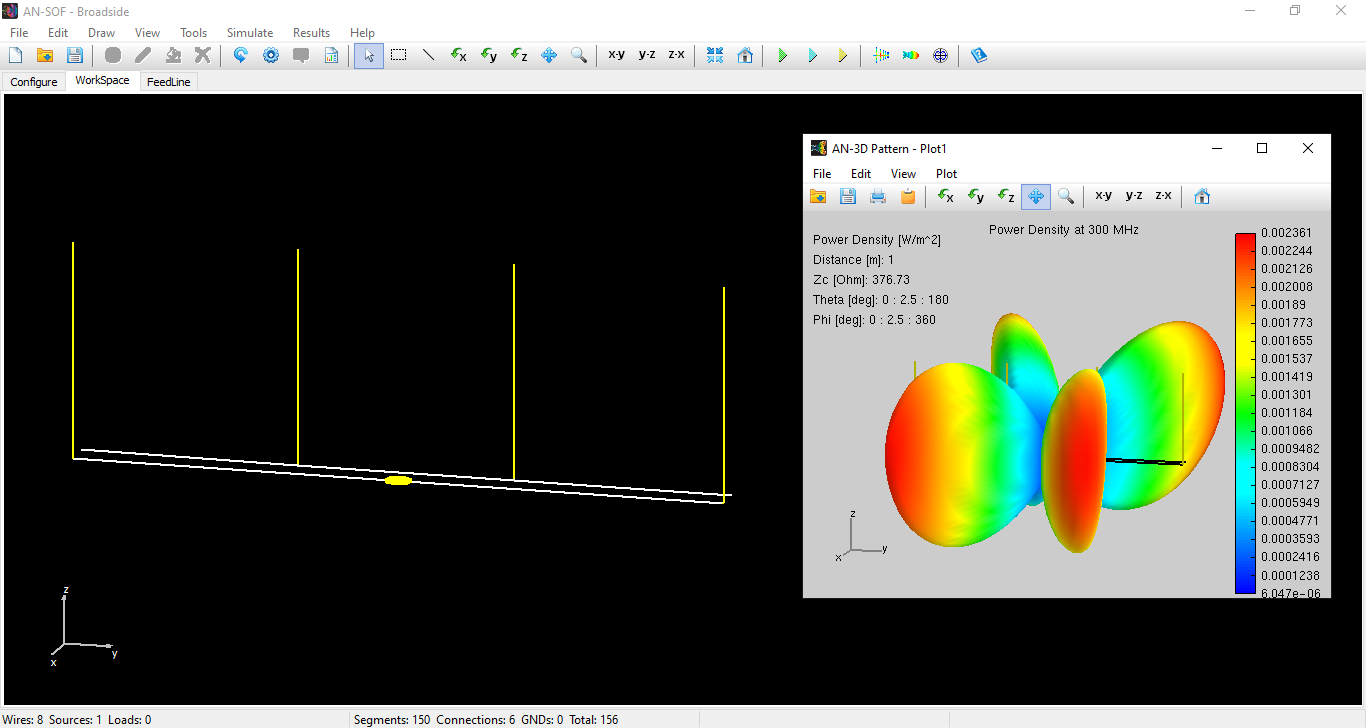Broadside antenna array composed of four parallel half-wave dipoles.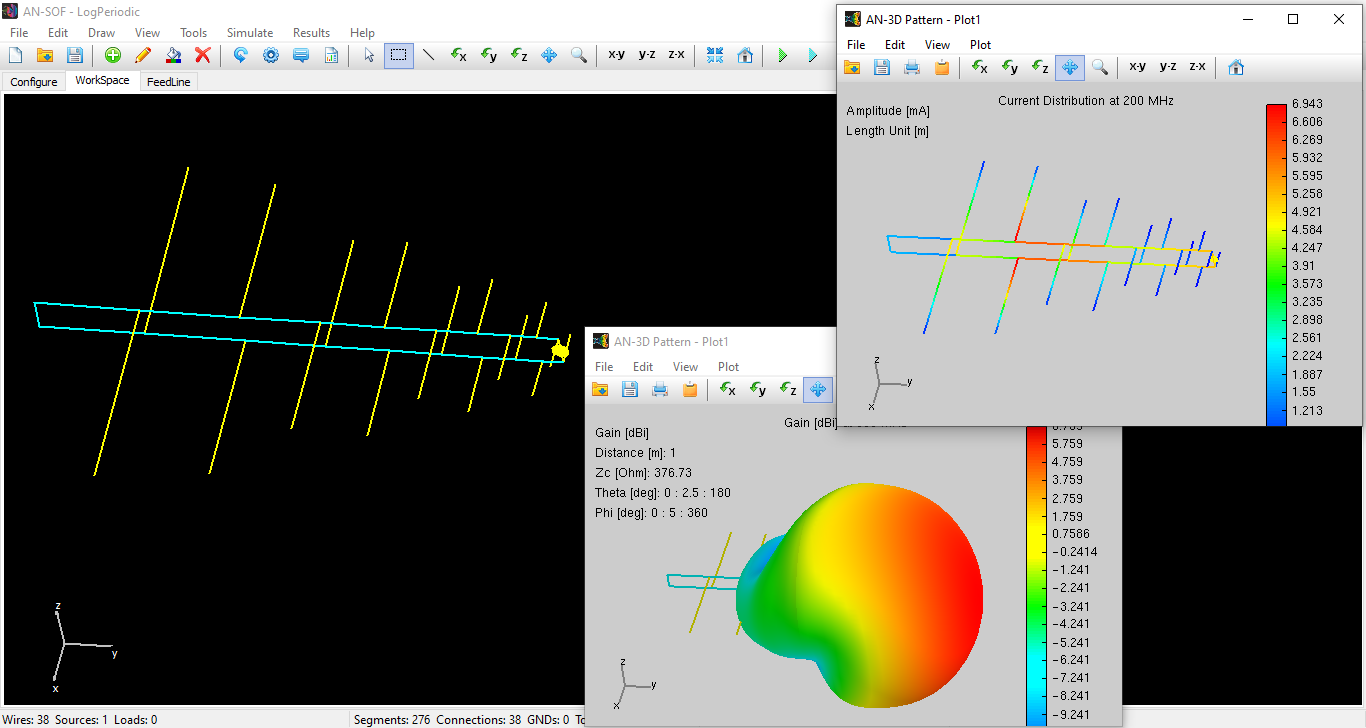## Log-Periodic Dipole Array

Frequency sweep simulation of a log-periodic array of linear elements.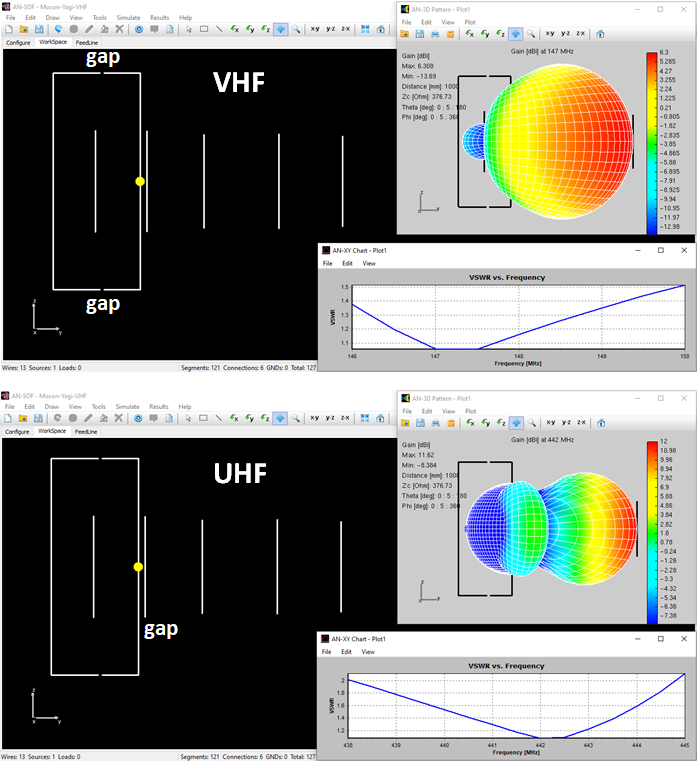## Moxon-Yagi Dual Band Antenna

Modeling a Moxon-Yagi Dual Band VHF/UHF Satellite Antenna.## 17m Band Spider Delta Loop

AN-SOF model of a 17 m Band Spider Delta Loop Antenna.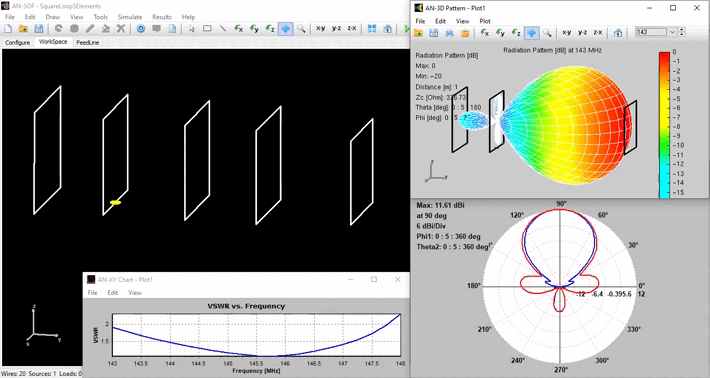## 145 MHz Array of Square Loops

Model of a 145 MHz 5-Element Array of Quads or Square Loops.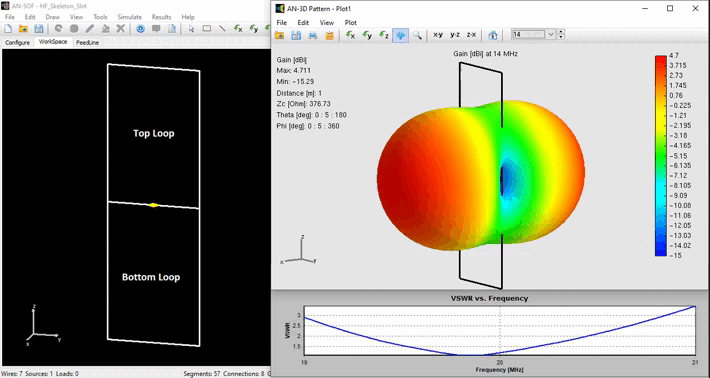## HF Skeleton Slot Antenna

The Skeleton Slot can be considered as an array of two tightly coupled loops. The image shows the top and bottom loops and the bi-directional pattern that is obtained.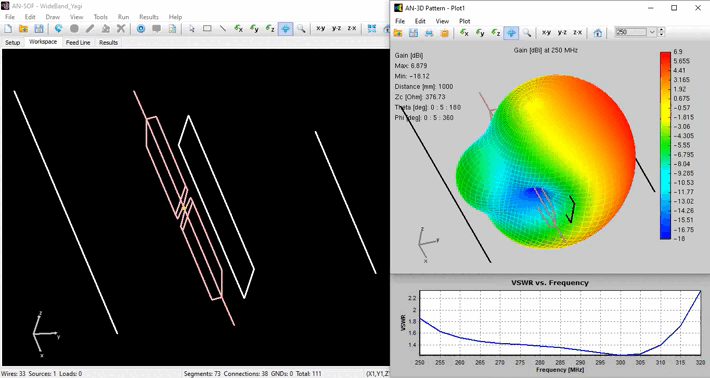This is a 4-element broadband directional antenna. More than 50 MHz of bandwidth (SWR < 1.5) around 285 MHz.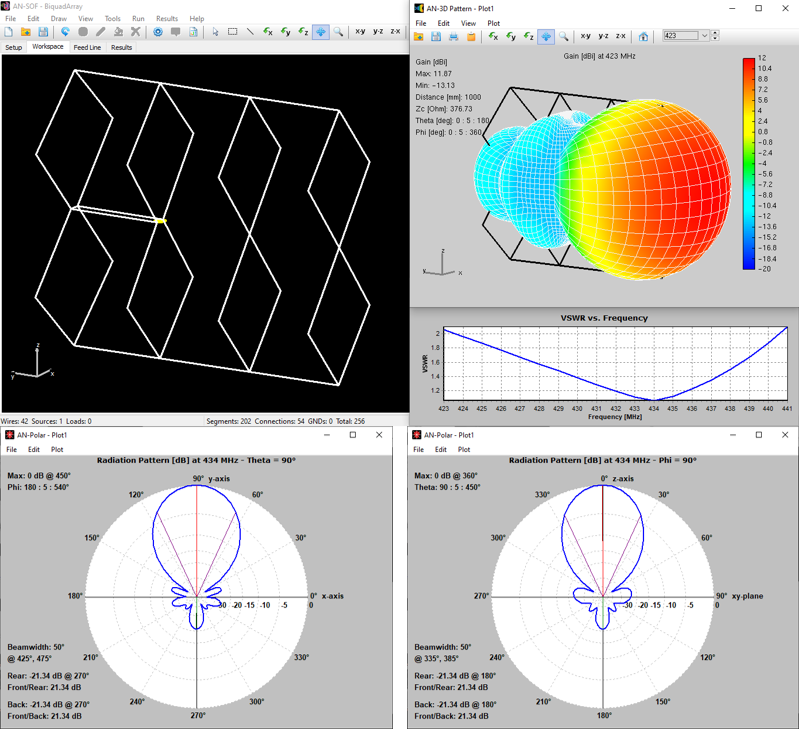This relatively compact 4-element biquad array resonates at 434 MHz.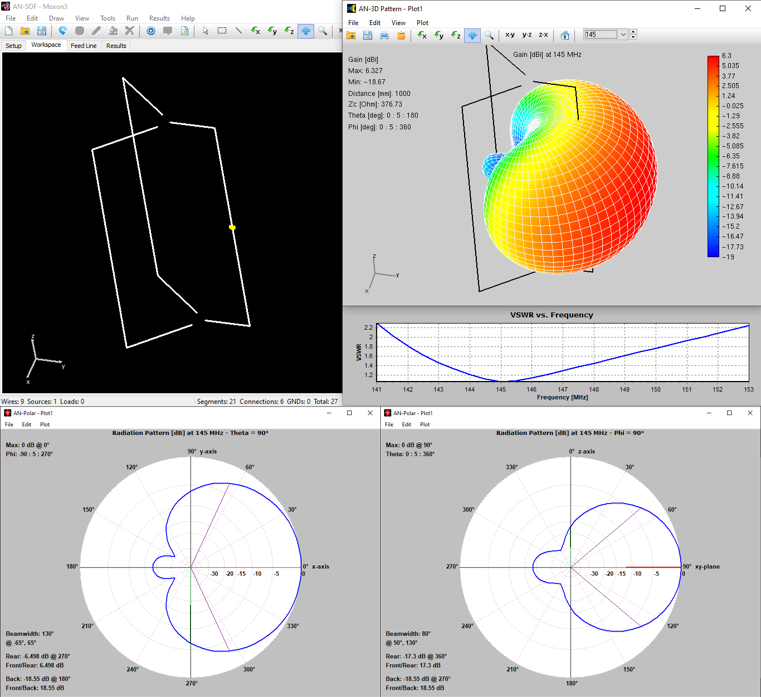## 145 MHz Moxon Antenna

This Moxon antenna variant resonates at 145 MHz. Input Impedance: 47 Ohm. Bandwidth: 7% (VSWR < 2). Gain: 6.3 dBi.## Horn Antenna

Simulation of a horn antenna fed by a rectangular waveguide.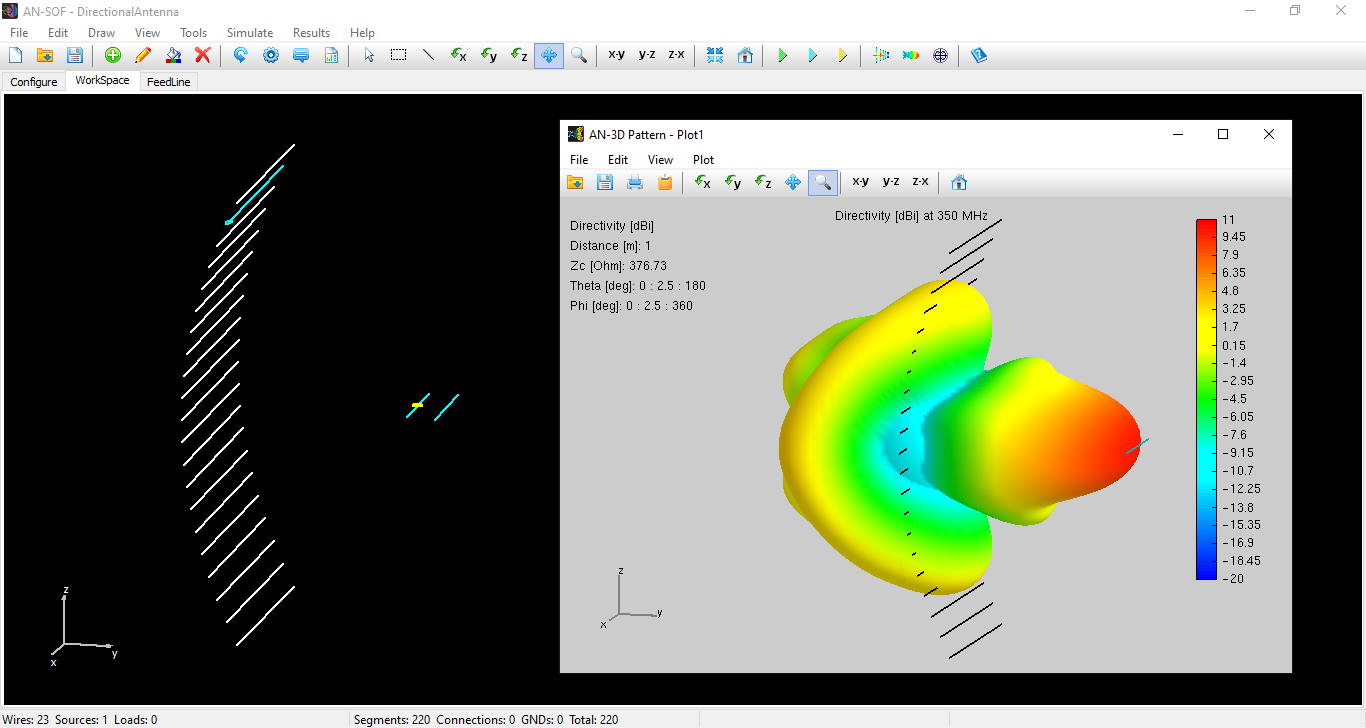## Parabolic Grid Antenna

Directional antenna consisting of a parabolic grid reflector and a Yagi-like array at the location of the parabola's focus.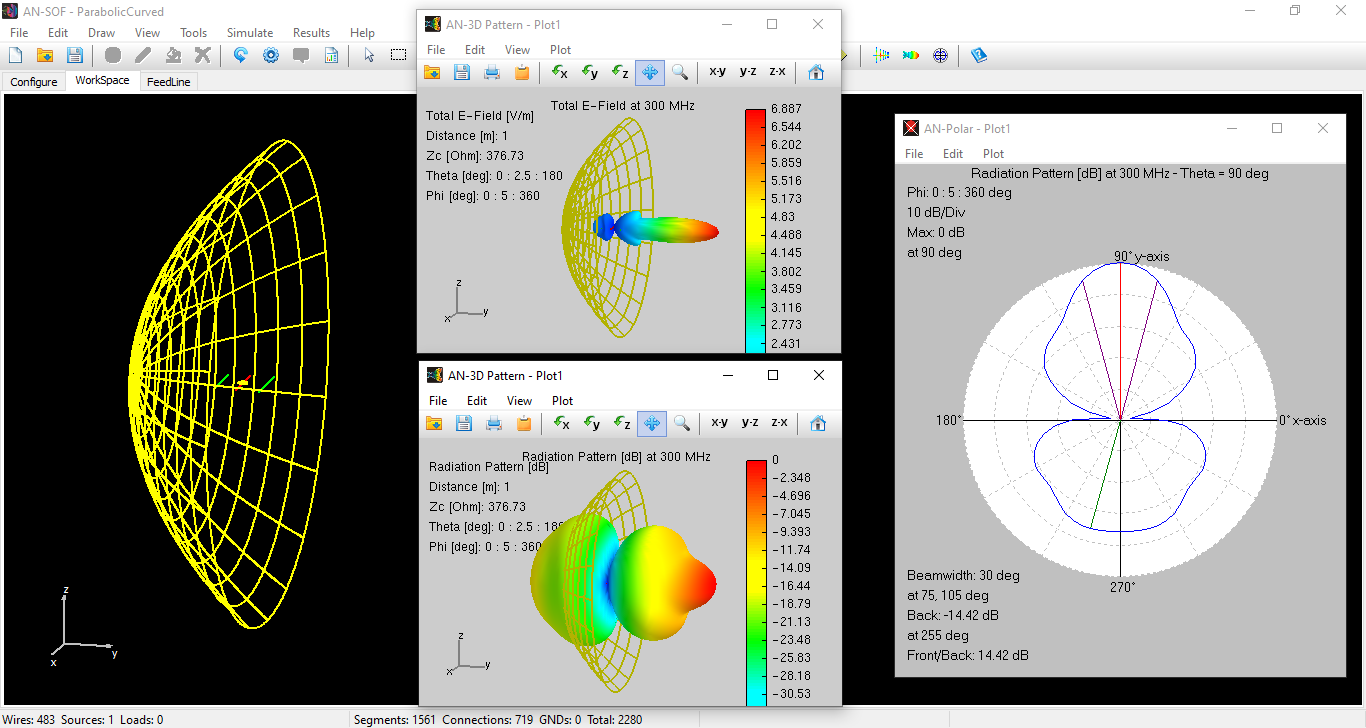## Dish Antenna

The reflector is modeled by a grid of curved segments. The hole sizes are small compared to the wavelength near the center of the parabola, buy they approach half-wavelength away from the center.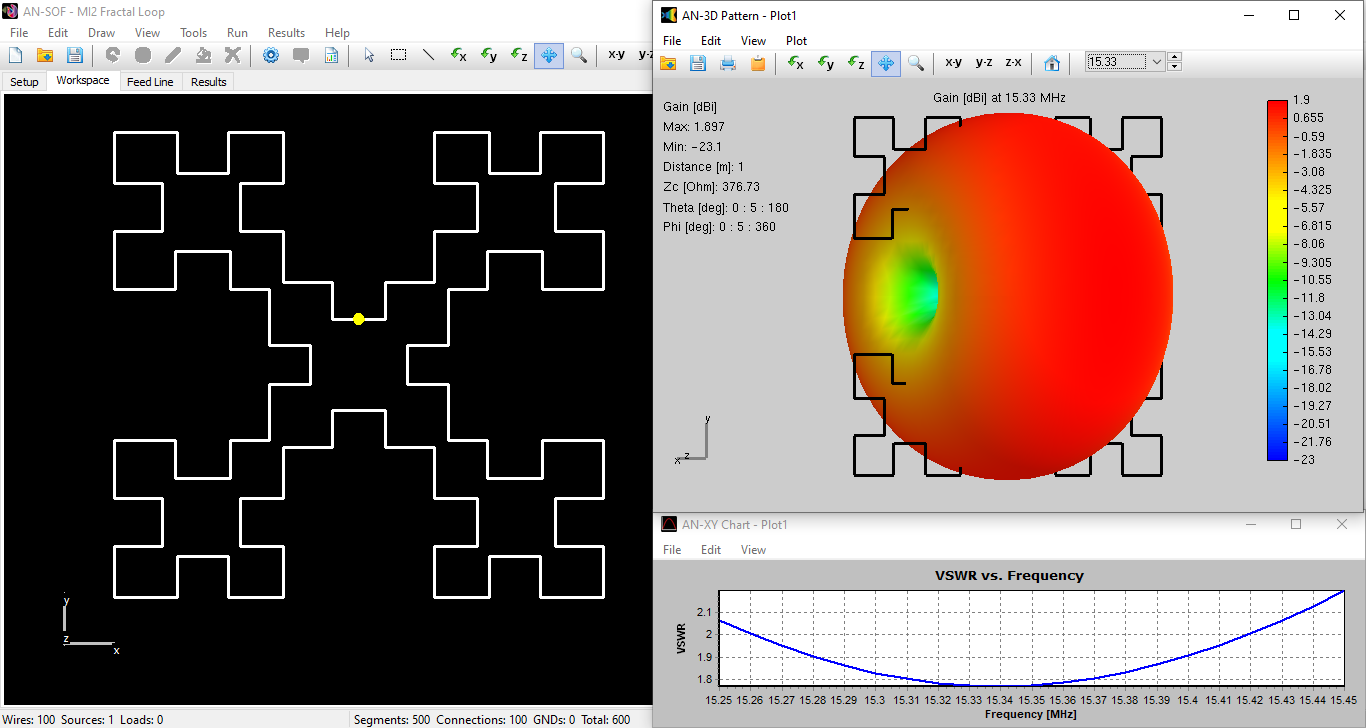## MI2 Fractal Loop

This MI2 Fractal Loop antenna was designed by Dr. Nathan Cohen. Results were reported in Cohen, N. L., and Hohlfeld, R. G., "Fractal Loops and the Small Loop Approximation", Communications Quarterly, 6, 77-81, (1996).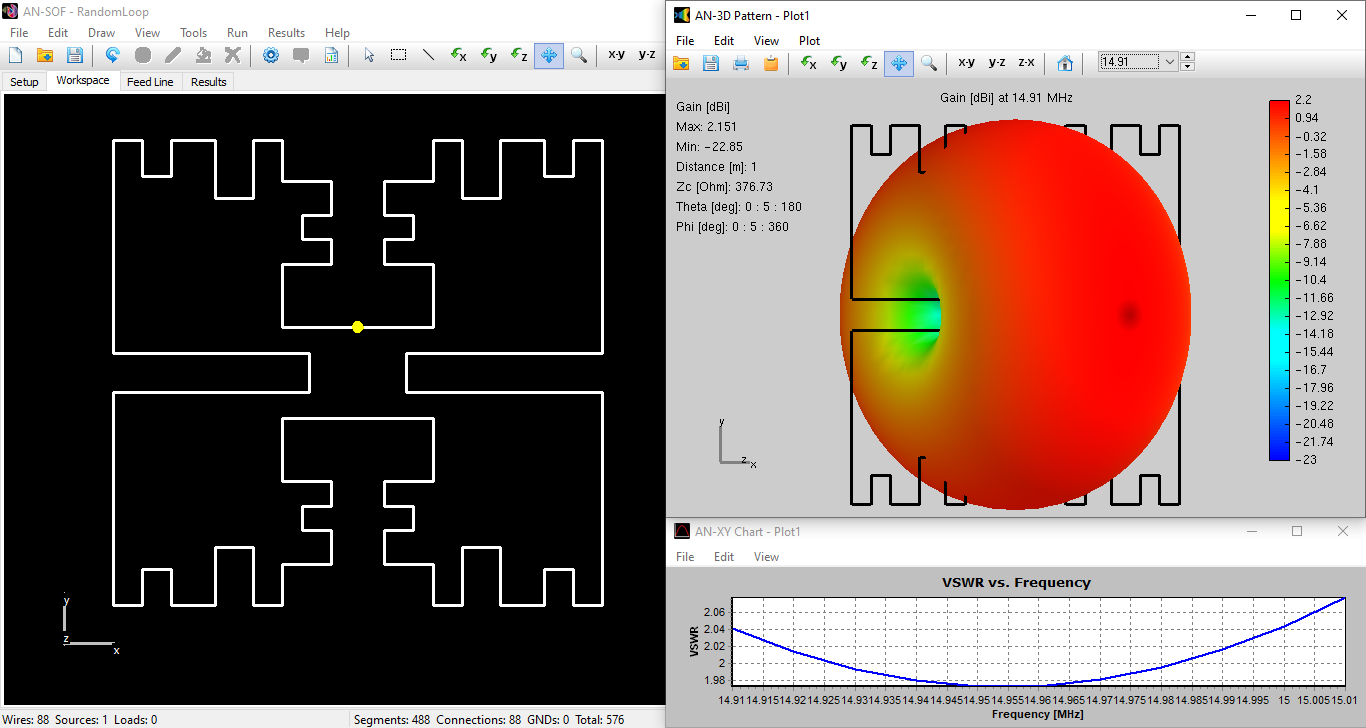## Random Loop

This random loop antenna was designed by VE9SRB in an attempt to demonstrate that a MI2 Fractal Loop-like performance can be obtained with an arbitrarily shaped geometry.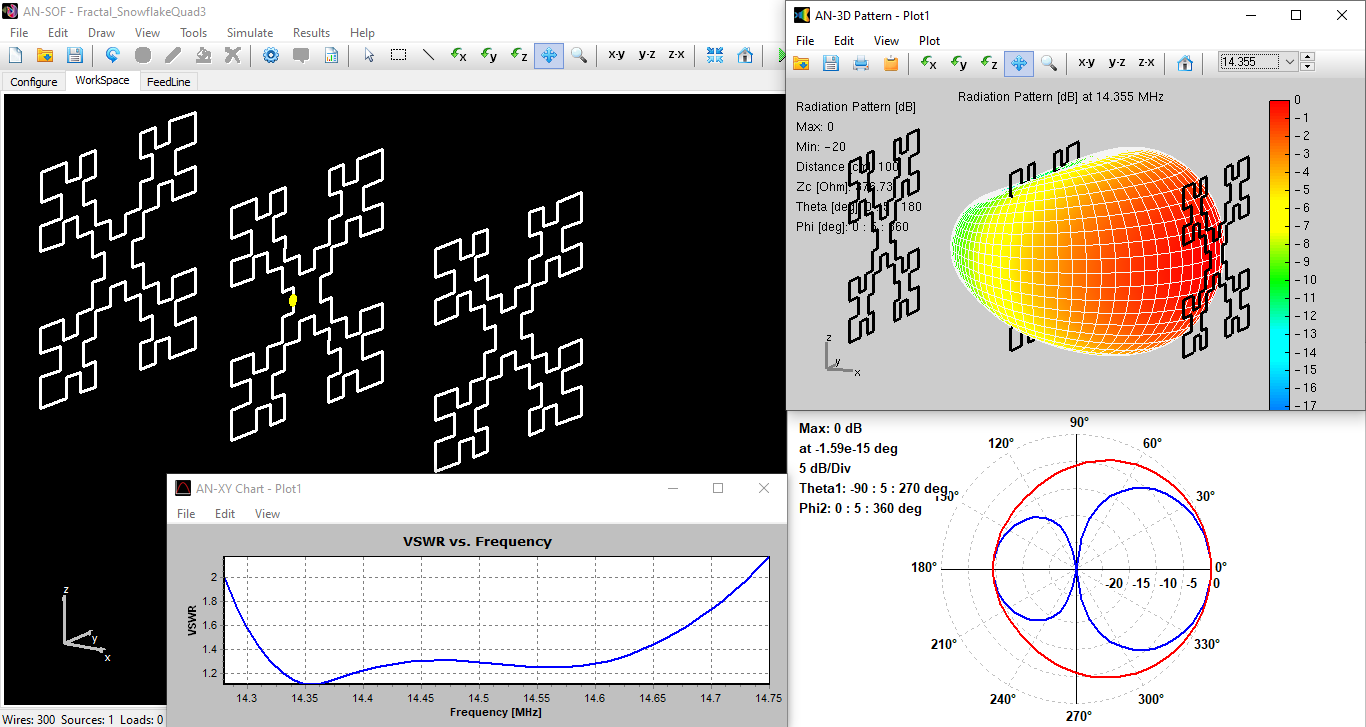Nathan Cohen in the US fractalized the quad loop based on the Minkowski square and invented an array of two elements. The biggest advantage of fractal antennas is that we get a wide bandwidth with a small size. This simulation shows that we can almost double the bandwidth with a 3-element array.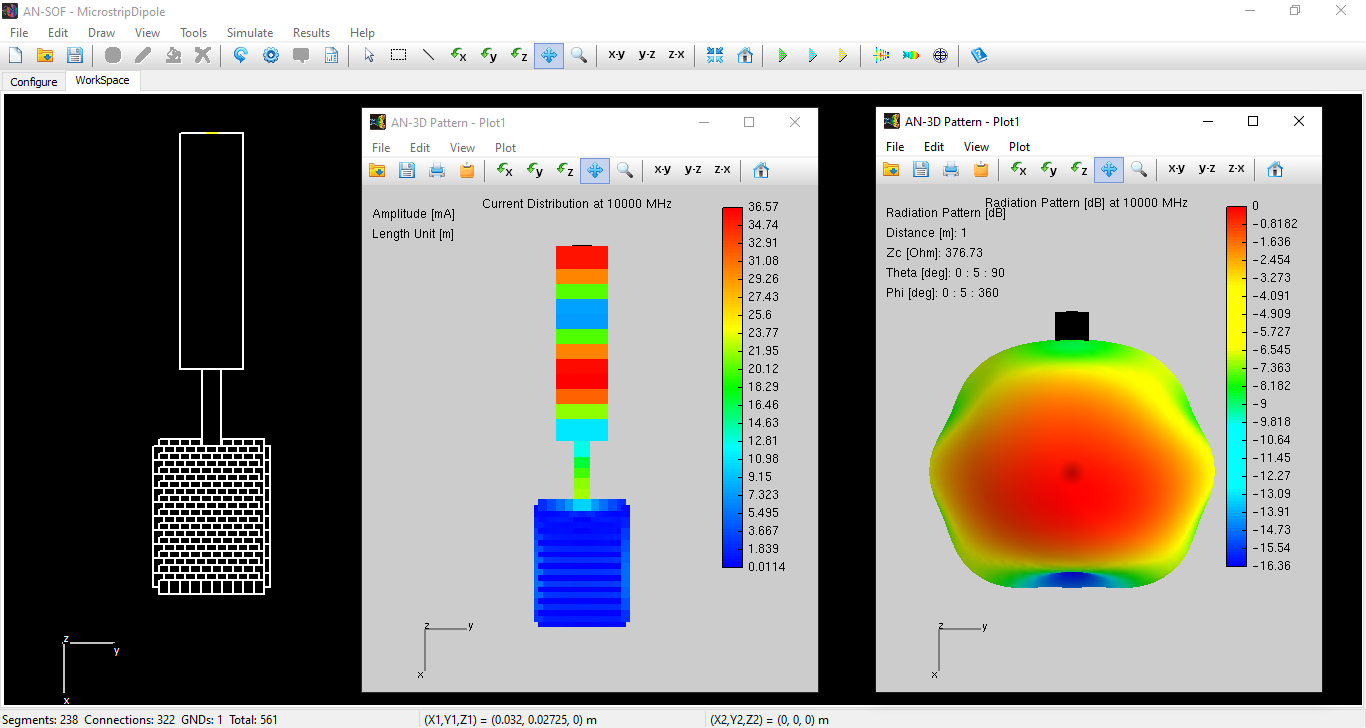## Microstrip Dipole

Microstrip patch dipole antenna over a dielectric substrate and backed by a perfect ground plane.## Patch Antenna

Microstrip patch antenna over a dielectric substrate and backed by a perfect ground plane.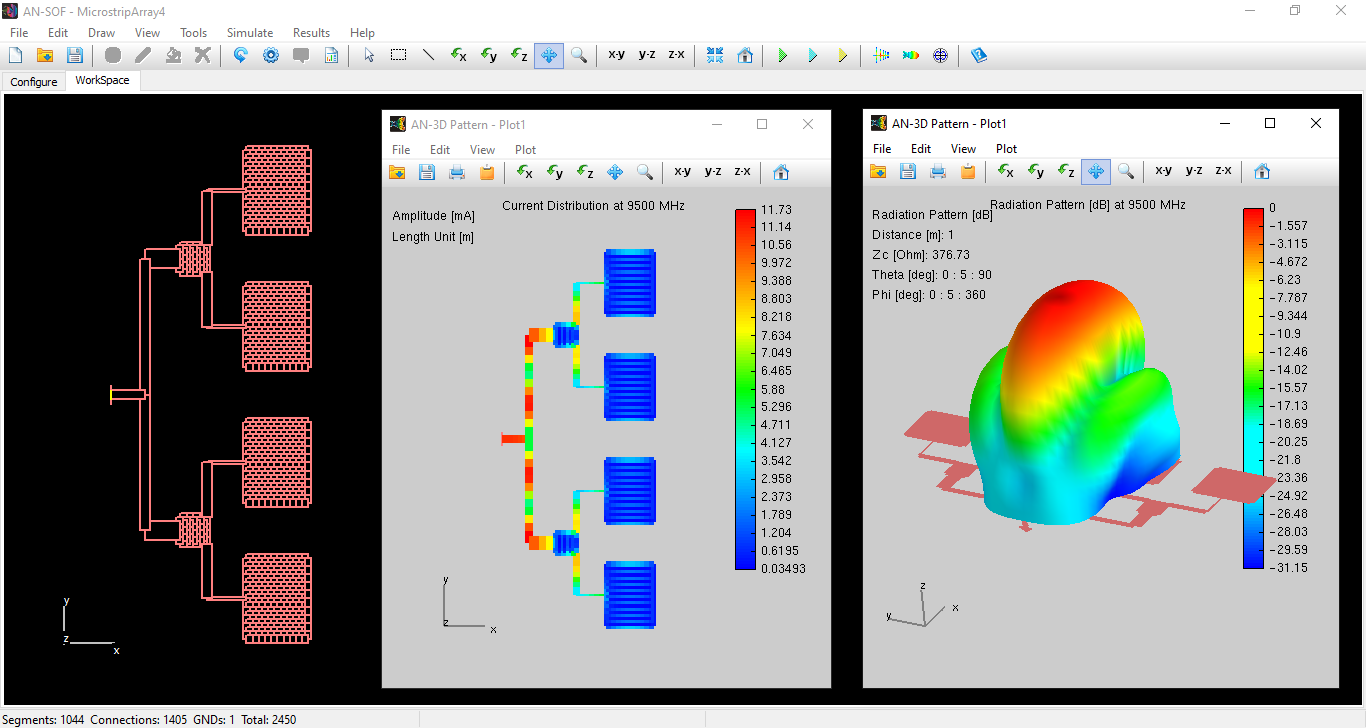## Microstrip Antenna Array

Microstrip array of four rectangular patches over a dielectric substrate and backed by a perfect ground plane.## Capacitively-Fed Patch

Microstrip patch antennas with capacitive feeding have been key in the development of the cell phone industry. This way of feeding compact antennas, for instance PIFAs, has made it possible to cancel out the probe reactance and simplify the matching network.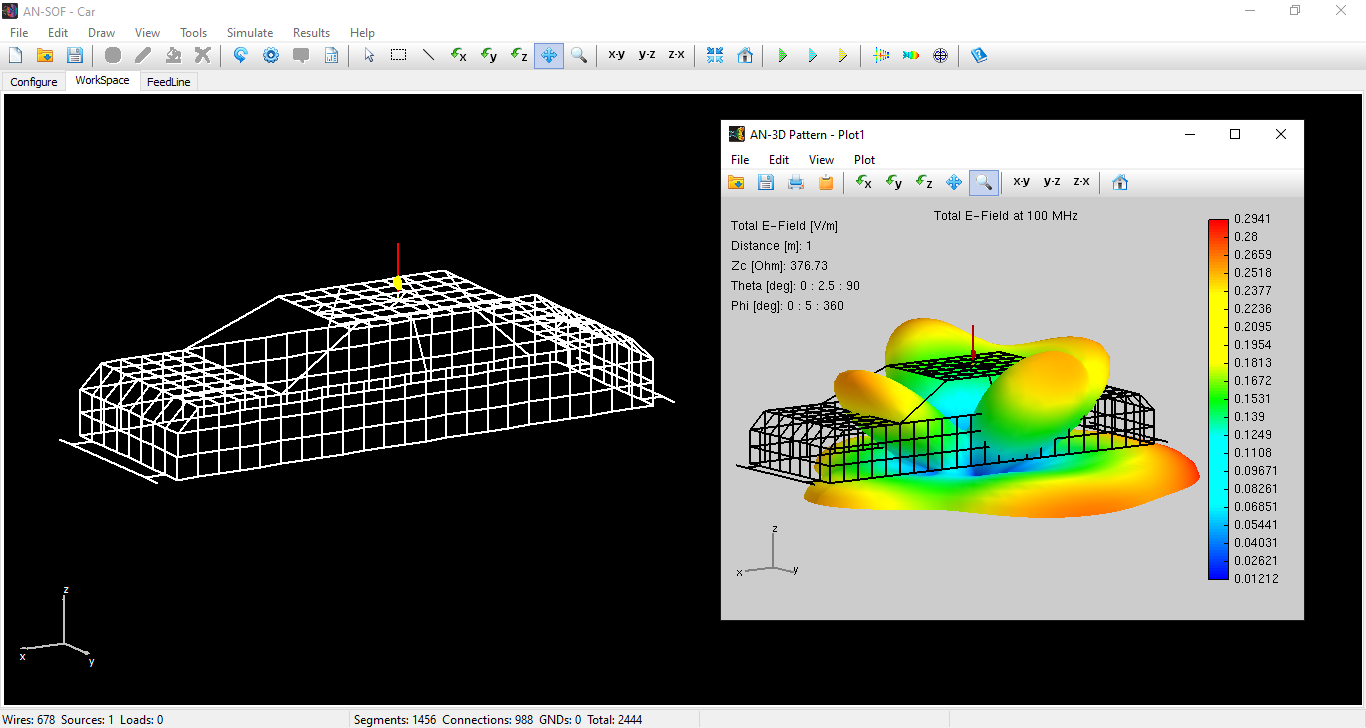## Car Roof Antenna

Simulation of a car above ground having a dipole antenna on its roof.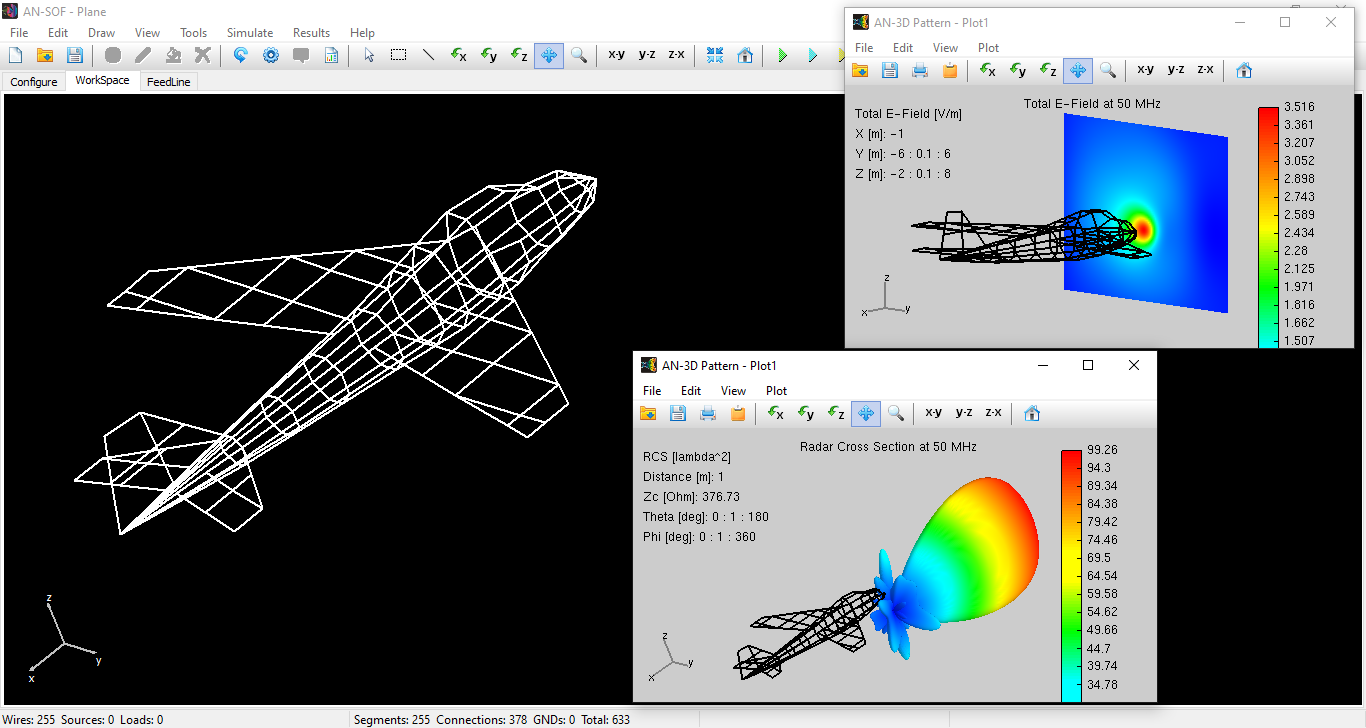## RCS of Aircraft

Simulation of a plane wave reaching the path of an airplane.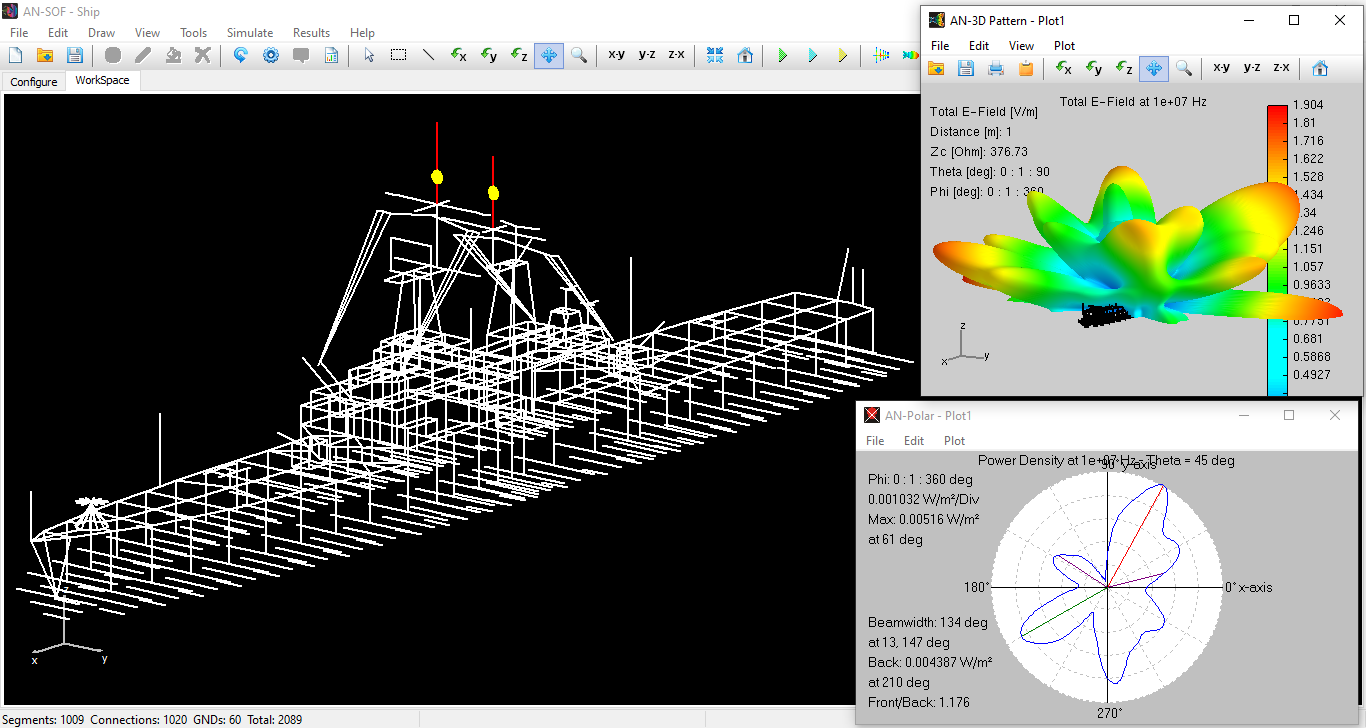## Antennas on a Ship

This simulation shows a wire grid model of a ship sailing in the ocean and having two dipole antennas at its top.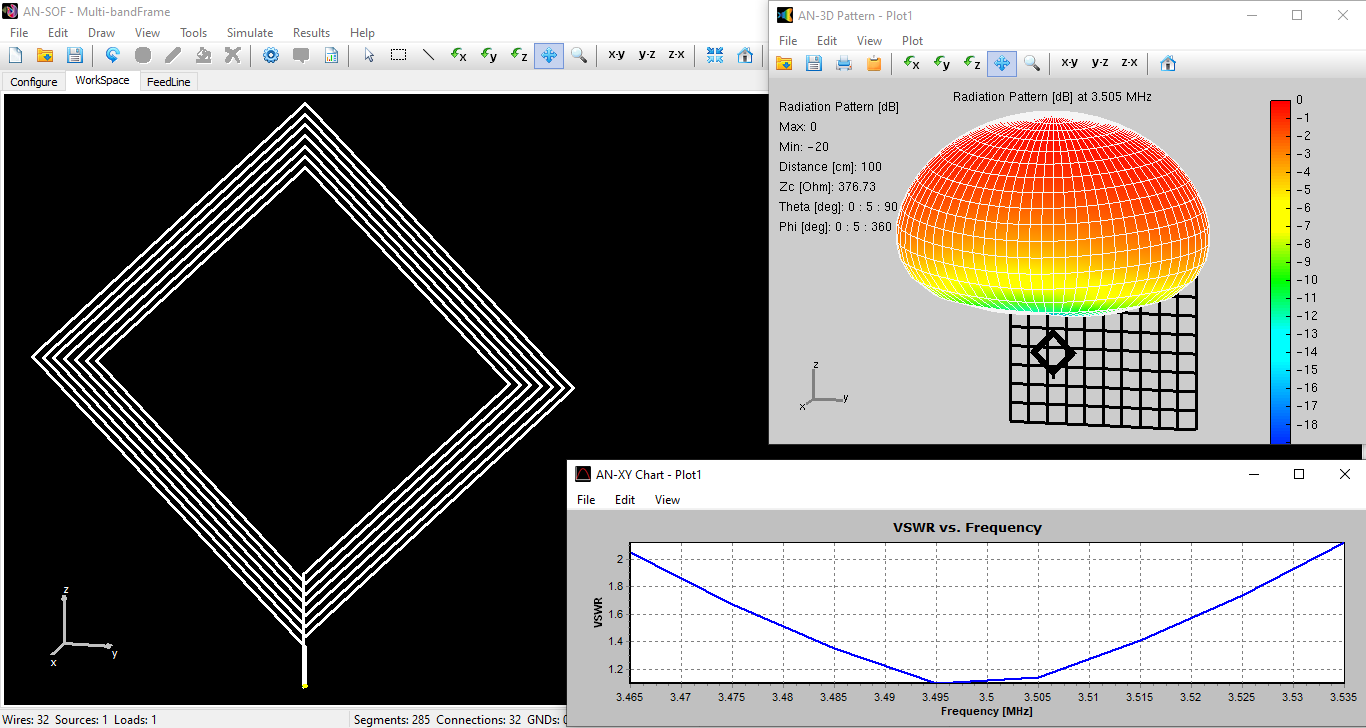## 80m DX Spiral Loop

A half-wave dipole would have a length of 40 meters in this band (3.75 MHz), difficult to install at home due to lack of space. A spiral loop is attractive for its small size and relative ease of tuning because it is basically an inductor to which a variable capacitor is connected at the feed point to achieve resonance.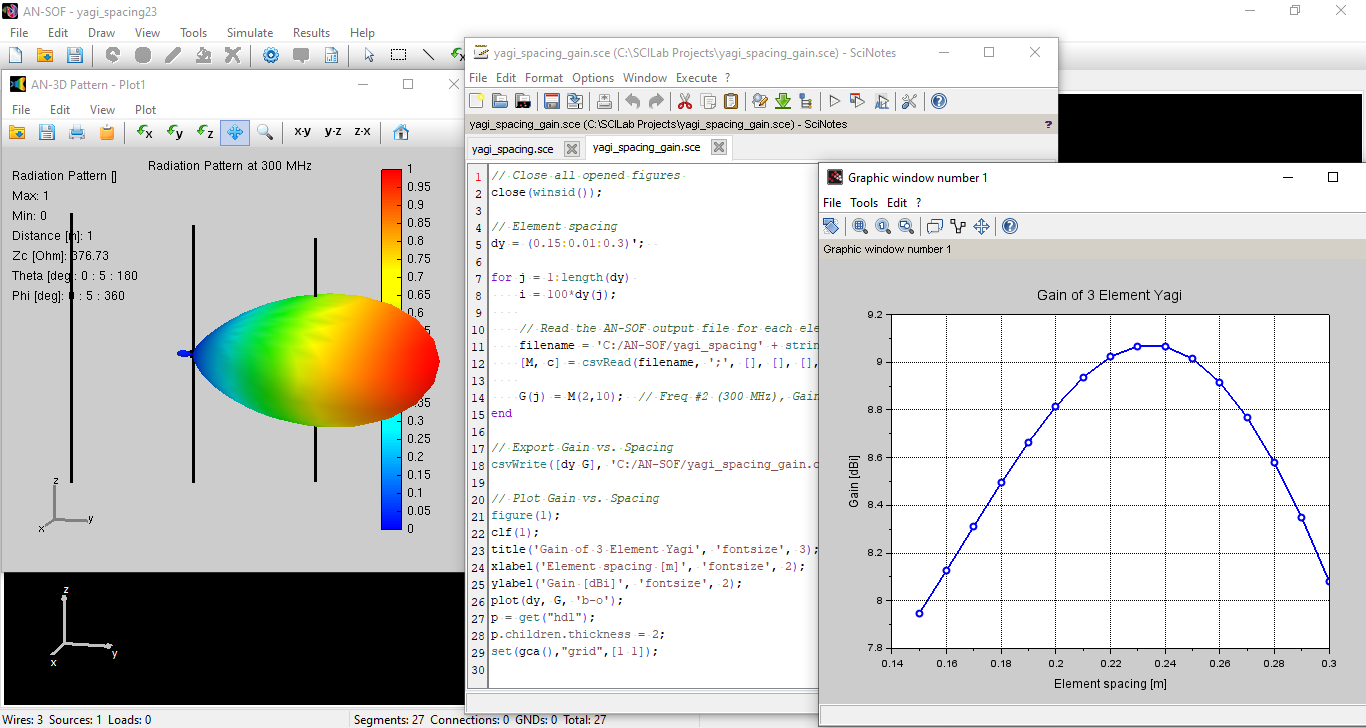## Element Spacing Simulation Script for Yagi-Uda Antennas

This guide explains how to run a script in Scilab to simulate a 3-element Yagi-Uda antenna and get the results as a function of the spacing between the elements. A second script allows us to plot the antenna gain versus element spacing.